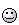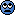# Magnitude of the electric field at equator (measured in kV)

Petra de Ruyter
Hi there

Only known variables include the solar flux on the Earths atmosphere at the equator is 1.5 kWm^ -2.

I've calculated the total energy from the Sun as 4 x 10^26 W, but can't seem to get to a calc of the mag of electric field at equator (measured in kVm^-2).

Gold Member
2021 Award
Hi there

Only known variables include the solar flux on the Earths atmosphere at the equator is 1.5 kWm^ -2.

I've calculated the total energy from the Sun as 4 x 10^26 W, but can't seem to get to a calc of the mag of electric field at equator (measured in kVm^-2).

I don't think you are going down the right path

the 1.5 kW m2 is the amount of sunlight which isn't going to help much in calculating the natural electric field of the Earth

have a look here for some ideas …..

https://encyclopedia2.thefreedictionary.com/Electric+Field+of+the+Earth

Dave

Petra de Ruyter
Is there a formula? A few websites state that it is 1.06 kVm^-1, however they do not say how it is calculated.

Gold Member
Is there a formula? A few websites state that it is 1.06 kVm^-1, however they do not say how it is calculated.
Which website? That figure suggests that we would all be electrocuted just by standing up!

•davenn
Homework Helper
Gold Member
suggests that we would all be electrocuted just by standing up!
You're sure? Positive? Absolutely certain?•davenn
Gold Member
You're sure? Positive? Absolutely certain?Not necessarily - the source resistance would be very high and our bodies would conduct any unbalanced charge and the potential at our head would be the potential of the nearby ground. However, I think there would have to be consequences which aren't so easily explained away.
But a field of 1kV/m would affect the ionosphere such that the + and - ions would form two layers, top and bottom of a layer, I think. Wouldn't that have been measured?
I'll wait until there's a proper reference about the idea.

Homework Helper
Gold Member
1.06 kVm^-1

willem2
Is there a formula? A few websites state that it is 1.06 kVm^-1, however they do not say how it is calculated.

I don't know where you found that number. At the surface of the earth there is an electric field of 100 to 150 V/m, due to the fact that the earth has a negative charge, and the ionosphere a positive charge.
See this link for example: http://www.feynmanlectures.caltech.edu/II_09.html

Petra de Ruyter
Greetings the source is in the book "The Light Fantastic: A modern introduction to Classical and Quantum Optics.

The answer to the question is listed as 1.06 kVm^-1 but does not state how this is calculated, I need some guidance as to how this figure was reached?

Homework Helper
Gold Member
how this figure was reached?
Direct measurement; 1940 s to 50 s? Thereabouts. Try the stacks in the library.

willem2
Greetings the source is in the book "The Light Fantastic: A modern introduction to Classical and Quantum Optics.

The answer to the question is listed as 1.06 kVm^-1 but does not state how this is calculated, I need some guidance as to how this figure was reached?
I think you are to use the formula given for the average energy density of a plane wave. (1.20)
$$N = \frac {\epsilon_{0}E^2c}{2}$$ where N is the average energy density and E the amplitude of the electric field of the wave.
Of course the light is incoherent, and the amplitude and direction of the field should vary randomly. Calculating an RMS value should come out the same. I don't think you have to prove that for the first exercise of the first chapter.

Petra de Ruyter
Greetings

Many thanks willem2. In my book it is (1.19). I appreciate all your help on this. I wont be doing this unit yet, but I wanted to get a head start on it.

cheers